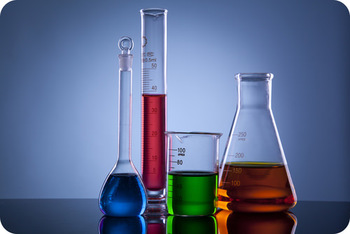Concentration of a solutionThe amount of substance present in a definite quantity of the solution is called concentration. A solution of known concentration is known as standard solution. And the solution of unknown concentration is known as unknown solution.

Concentration of a solution is usually expressed in terms of normality or molarity in volumetric analysis. However, the concentration can be expressed in terms of percentage, gm per litre, formality and mole fraction besides normality and molarity. Different concentration forms are discussed below:

1. Percentage Solution
It is defined as the number of parts of the substance present in 100 parts of the substance present in 100 parts of the solution. e.g. v/v ( volume / volume ), w/v ( weight / volume ), w/w ( weight / weight ) are usually indicated to percentage solution.

For example,
-> 5% NaCl solution ( w/v ) indicates that 5 g of NaCl is present in 100 ml of its solution.
-> 10% NaOH solution  ( w/w ) indicates that 10 g of NaOH is present in 100 g of its solution.
-> 15% acetone solution ( v/v ) indicates that 15 ml of acetone is present in 100 ml of its solution.

2. Gram per litre solution
It is defined as the amount of substance in gram present in a litre of its solution. For example, 5g per litre NaOH solution indicates that 5 g of NaOH is pressent in 1 litre ( 1000 ml ) of its solution.

3. Normality

It is defined as the number of gram equivalents of the substance dissolved in a litre of its solution. It is denoted by N.

Mathematically,

Normality =                 No. of gram equivalent of solute
Volume of solution in litre

No. of gram equivalent =            Weight of solute in gram
Equivalent weight of solute

Thus,

Normality =                              Weight of solute in gm
Equivalent weight of solute * Volume of solution in ml

=                              Weight of solute in gm * 1000
Equivalent weight of solute * Volume of solution in litre

4. Molarity

It is defined as the number of gram molecular weight ( moles ) of a substance dissolved in a litre of its solution. It is denoted by M.

Mathematically,

Molarity =              No. of gram moles of solute
Volume of solution in litres

But number of moles =               Weight of solute in gm
Molecular weight in gram

Therefore,

Molarity =                     Weight of solute in gm * 1000
Molecular wt. of solute * Volume of solution in ml

5.Molality
It is defined as the number of moles of the substance dissolved in 1 kg ( 1000 g ) of solvent. It is denoted by 'm'

Mathematically,

Molality =               No. of moles of substance               * 1000
Weight of solvent in gm

=                    Weight of substance in gram * 1000
Molecular weight of solute * weight of solvent in gram

6. Formality

It is defined as the number of gram formula weights of the ionic solute dissolved in one litre of the solution. It is represented by F. i.e.

Formality ( F ) =              Number of gram formula weight
Volume of solution in litre

*Commonly formality term is used to express the concentration of the ionic solids which do not exist as molecules.

7. Mole fraction

Mole fraction can be defined as the ratio of number of moles of one component to the total number of moles of all the components ( solvent & solute ) present in solution. Let, us suppose that a solution contains 'n' moles of a solute in 'N' moles of solvent. Then,

Total number of moles present in the solution = n + N

Thus,

The mole fraction of solute (X solute ) =         n
n + N

and mole fraction of solvent ( X solvent ) =         N
n + n

The sum of mole fractions of all the component is always one.

i.e. X solute + X solvent =        n         +         N         =   1
n + N             n + N